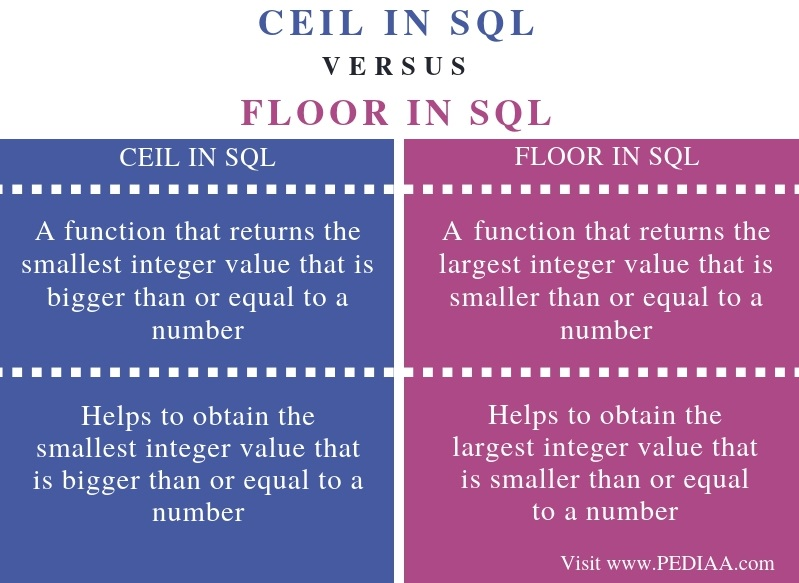What is the Difference Between CEIL and FLOOR in SQL

The main difference between CEIL and FLOOR in SQL is that CEIL helps to obtain the smallest integer value that is bigger than or equal to a number while FLOOR helps to obtain the largest integer value that is smaller than or equal to a number.

A Database Management System (DBMS) is a software that helps to store and manage data in databases. A DBMS that follows the relational model is a Relational DBMS (RDBMS). There are various RDBMS such as MySQL. The programmer can execute a Structured Query Language (SQL) to perform operations on the data in the databases. There are several SQL functions, and CEIL and FLOOR are two of them.

Key Areas Covered

1. What is CEIL in SQL
– Definition, Functionality
2. What is FLOOR in SQL
– Definition, Functionality
3. Difference Between CEIL and FLOOR in SQL
– Comparison of Key Differences

Key Terms

CEIL, FLOOR, RDBMS, SQLWhat is CEIL in SQL

The CEIL() function helps to return the smallest integer value that is bigger than or equal to a specific number.The syntax is as follows.

CEIL (number)

An example is as follows.

SELECT CEIL(50.87);

It returns 51 as the output.

Another example is as follows.

SELECT CEIL (60.12);

It returns 61 as the output.

What is Floor in SQL

The FLOOR () function helps to return the largest integer value that is smaller than or equal to a specific number.  The syntax is as follows.

FLOOR (number)

An example is as follows.

SELECT FLOOR (50.87);

It returns 50 as the output.

Another example is as follows.

SELECT FLOOR (60.12);

It returns 60 as the output.

Difference Between CEIL and FLOOR in SQL

Definition

CEIL() is a function that returns the smallest integer value that is bigger than or equal to a number while FLOOR() is a function that returns the largest integer value that is smaller than or equal to a number. Thus, this is the main difference between CEIL and FLOOR in SQL.

Usage

Another difference between CEIL and FLOOR in SQL is that CEIL() helps to obtain the smallest integer value that is bigger than or equal to a number. FLOOR() helps to obtain the largest integer value that is smaller than or equal to a number.

Conclusion

RDBMS such as MySQL allows the programmers to write SQL queries to perform operations on the data stored in the databases. Two such functions are CEIL and FLOOR. The main difference between CEIL and FLOOR in SQL is that CEIL() helps to obtain the smallest integer value that is bigger than or equal to a number while FLOOR() helps to obtain the largest integer value that is smaller than or equal to a number.

Reference

1. “MySQL CEIL() Function”, w3schools.com, Available here.
2. “MySQL FLOOR() Function”, w3schools.com, Available here.

Image Courtesy:

1. “2394312” (CC0) via Pixabay